### Program Statements

This section lists the program statements used to code the objective function and nonlinear constraints and their derivatives, and it documents the differences between program statements in the NLP procedure and program statements in the DATA step. The syntax of program statements used in PROC NLP is identical to that used in the CALIS, GENMOD, and MODEL procedures (refer to the SAS/ETS User’s Guide).

Most of the program statements which can be used in the SAS DATA step can also be used in the NLP procedure. See the SAS Language Guide or base SAS documentation for a description of the SAS program statements.

 `ABORT;` `CALL` name [ ( expression [, expression …] ) ]; `DELETE;` `DO` [ variable `=` expression [ `TO` expression ] [ `BY` expression ] [, expression [ `TO` expression ] [ `BY` expression ] …] ] [ `WHILE` expression ] [ `UNTIL` expression ]; `END;` `GOTO` statement_label; `IF` expression; `IF` expression `THEN` program_statement; `ELSE` program_statement; variable `=` expression; variable `+` expression; `LINK` statement_label; `PUT` [ variable] [=] [...]; `RETURN`; `SELECT [(` expression `)]`; `STOP`; `SUBSTR(` variable, index, length `) =` expression; `WHEN (` expression`)` program_statement; `OTHERWISE` program_statement;

For the most part, the SAS program statements work as they do in the SAS DATA step as documented in the SAS Language Guide. However, there are several differences that should be noted.

• The ABORT statement does not allow any arguments.

• The DO statement does not allow a character index variable. Thus

`do i = 1,2,3;`

is supported; however,

`do i = ’A’,’B’,’C’;`

is not.

• The PUT statement, used mostly for program debugging in PROC NLP, supports only some of the features of the DATA step PUT statement, and has some new features that the DATA step PUT statement does not:

• The PROC NLP PUT statement does not support line pointers, factored lists, iteration factors, overprinting, _INFILE_, the colon (:) format modifier, or \$.

• The PROC NLP PUT statement does support expressions, but the expression must be enclosed inside of parentheses. For example, the following statement displays the square root of x:  `put (sqrt(x));`

• The PROC NLP PUT statement supports the print item _PDV_ to print a formatted listing of all variables in the program. For example, the following statement displays a more readable listing of the variables than the _all_ print item:  `put _pdv_;`

• The WHEN and OTHERWISE statements allow more than one target statement. That is, DO/END groups are not necessary for multiple statement WHENs. For example, the following syntax is valid:

 `SELECT;` `WHEN (` exp1 `)` stmt1; stmt2; `WHEN (` exp2 `)` stmt3; stmt4; `END;`

It is recommended to keep some kind of order in the input of NLP, that is, between the statements that define decision variables and constraints and the program code used to specify objective functions and derivatives.

#### Use of Special Variables in Program Code

Except for the quadratic programming techniques (QUADAS and LICOMP) that do not execute program statements during the iteration process, several special variables in the program code can be used to communicate with PROC NLP in special situations:

• _OBS_ If a DATA= input data set is used, it is possible to access a variable _OBS_ which contains the number of the observation processed from the data set. You should not change the content of the _OBS_ variable. This variable enables you to modify the programming statements depending on the observation number processed in the DATA= input data set. For example, to set variable A to 1 when observation 10 is processed, and otherwise to 2, it is possible to specify

```       IF _OBS_ = 10 THEN A=1; ELSE A=2;
```
• _ITER_ This variable is set by PROC NLP, and it contains the number of the current iteration of the optimization technique as it is displayed in the optimization history. You should not change the content of the _ITER_ variable. It is possible to read the value of this variable in order to modify the programming statements depending on the iteration number processed. For example, to display the content of the variables A, B, and C when there are more than 100 iterations processed, it is possible to use

```      IF _ITER_ > 100 THEN PUT A B C;
```
• _DPROC_ This variable is set by PROC NLP to indicate whether the code is called only to obtain the values of theobjective functions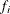(_DPROC_=0) or whether specified derivatives (defined by the GRADIENT, JACOBIAN, CRPJAC, or HESSIAN statement) also have to be computed (_DPROC_=1). You should not change the content of the _DPROC_ variable. Checking the _DPROC_ variable makes it possible to save computer time by not performing derivative code that is not needed by the current call. In particular, when a DATA= input data set is used, the code is processed many times to compute only the function values. If the programming statements in the program contain the specification of computationally expensive first- and second-order derivatives, you can put the derivative code in an IF statement that is processed only if _DPROC_ is not zero.

• _INDF_ The _INDF_ variable is set by PROC NLP to inform you of the source of calls to the function or derivative programming.

_INDF_=0

indicates the first function call in a grid search. This is also the first call evaluating the programming statements if there is a grid search defined by grid values in the DECVAR statement.

_INDF_=1

indicates further function calls in a grid search.

_INDF_=2

indicates the call for the feasible starting point. This is also the first call evaluating the programming statements if there is no grid search defined.

_INDF_=3

indicates calls from a gradient-checking algorithm.

_INDF_=4

indicates calls from the minimization algorithm. The _ITER_ variable contains the iteration number.

_INDF_=5

If the active set algorithm leaves the feasible region (due to rounding errors), an algorithm tries to return it into the feasible region; _INDF_=5 indicates a call that is done when such a step is successful.

_INDF_=6

indicates calls from a factorial test subroutine that tests the neighborhood of a pointfor optimality.

_INDF_=7, 8

indicates calls from subroutines needed to compute finite-difference derivatives using only values of the objective function. No nonlinear constraints are evaluated.

_INDF_=9

indicates calls from subroutines needed to compute second-order finite-difference derivatives using analytic (specified) first-order derivatives. No nonlinear constraints are evaluated.

_INDF_=10

indicates calls where only the nonlinear constraints but no objective function are needed. The analytic derivatives of the nonlinear constraints are computed.

_INDF_=11

indicates calls where only the nonlinear constraints but no objective function are needed. The analytic derivatives of the nonlinear constraints are not computed.

_INDF_=-1

indicates the last call at the final solution.

You should not change the content of the _INDF_ variable.

• _LIST_ You can set the _LIST_ variable to control the output during the iteration process:

_LIST_=0

is equivalent to the NOPRINT option. It suppresses all output.

_LIST_=1

is equivalent to the PSUMMARY but not the PHISTORY option. The optimization start and termination messages are displayed. However, the PSUMMARY option suppresses the output of the iteration history.

_LIST_=2

is equivalent to the PSHORT option or to a combination of the PSUMMARY and PHISTORY options. The optimization start information, the iteration history, and termination message are displayed.

_LIST_=3

is equivalent to not PSUMMARY, not PSHORT, and not PALL. The optimization start information, the iteration history, and the termination message are displayed.

_LIST_=4

is equivalent to the PALL option. The extended optimization start information (also containing settings of termination criteria and other control parameters) is displayed.

_LIST_=5

In addition to the iteration history, the vector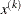of parameter estimates is displayed for each iteration.

_LIST_=6

In addition to the iteration history, the vectorof parameter estimates and the gradient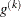(if available) of the objective function are displayed for each iteration.

It is possible to set the _LIST_ variable in the program code to obtain more or less output in each iteration of the optimization process. For example,

```      IF _ITER_ = 11      THEN _LIST_=5;
ELSE IF _ITER_ > 11 THEN _LIST_=1;
ELSE _LIST_=3;
```
• _TOOBIG_ The value of _TOOBIG_ is initialized to 0 by PROC NLP, but you can set it to 1 during the iteration, indicating problems evaluating the program statements. The objective function and derivatives must be computable at the starting point. However, during the iteration it is possible to set the _TOOBIG_ variable to 1, indicating that the programming statements (computing the value of the objective function or the specified derivatives) cannot be performed for the current value of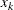. Some of the optimization techniques check the value of _TOOBIG_ and try to modify the parameter estimates so that the objective function (or derivatives) can be computed in a following trial.

• _NOBS_ The value of the _NOBS_ variable is initialized by PROC NLP to the product of the number of functions mfun specified in the MIN, MAX or LSQ statement and the number of valid observations nobs in the current BY group of the DATA= input data set. The value of the _NOBS_ variable is used for computing the scalar factor of the covariance matrix (see the COV=, VARDEF=, and SIGSQ= options). If you reset the value of the _NOBS_ variable, the value that is available at the end of the iteration is used by PROC NLP to compute the scalar factor of the covariance matrix.

• _DF_ The value of the _DF_ variable is initialized by PROC NLP to the numberof parameters specified in the DECVAR statement. The value of the _DF_ variable is used for computing the scalar factor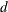of the covariance matrix (see the COV=, VARDEF=, and SIGSQ= options). If you reset the value of the _DF_ variable, the value that is available at the end of the iteration is used by PROC NLP to compute the scalar factor of the covariance matrix.

• _LASTF_ In each iteration (except the first one), the value of the _LASTF_ variable is set by PROC NLP to the final value of the objective function that was achieved during the last iteration. This value should agree with the value that is displayed in the iteration history and that is written in the OUTEST= data set when the OUTITER option is specified.